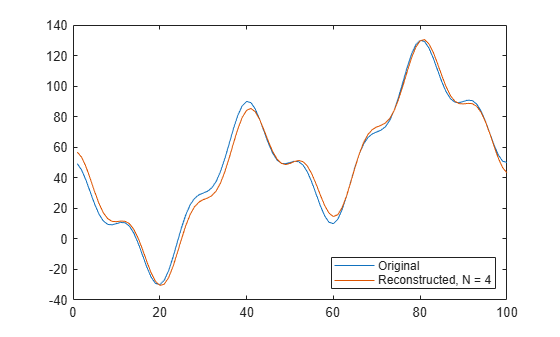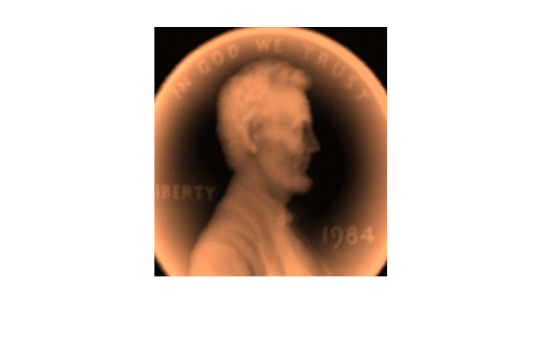dct

Discrete cosine transform

Description

example

y = dct(x) returns the unitary discrete cosine transform of input array x. The output y has the same size as x. If x has more than one dimension, then dct operates along the first array dimension with size greater than 1.

y = dct(x,n) zero-pads or truncates the relevant dimension of x to length n before transforming.

example

y = dct(x,n,dim) computes the transform along dimension dim. To input a dimension and use the default value of n, specify the second argument as empty, [].

example

y = dct(___,'Type',dcttype) specifies the type of discrete cosine transform to compute. See Discrete Cosine Transform for details. This option can be combined with any of the previous syntaxes.

Examples

collapse all

Find how many DCT coefficients represent 99% of the energy in a sequence.

x = (1:100) + 50*cos((1:100)*2*pi/40);
X = dct(x);
[XX,ind] = sort(abs(X),'descend');
i = 1;
while norm(X(ind(1:i)))/norm(X) < 0.99
i = i + 1;
end
needed = i;

Reconstruct the signal and compare it to the original signal.

X(ind(needed+1:end)) = 0;
xx = idct(X);

plot([x;xx]')
legend('Original',['Reconstructed, N = ' int2str(needed)], ...
'Location','SouthEast')Load a file that contains depth measurements of a mold used to mint a United States penny. The data, taken at the National Institute of Standards and Technology, are sampled on a 128-by-128 grid. Display the data.

surf(P)
view(2)
colormap copper
axis ij square offCompute the discrete cosine transform of the image data. Operate first along the rows and then along the columns.

Q = dct(P,[],1);
R = dct(Q,[],2);

Find what fraction of DCT coefficients contain 99.98% of the energy in the image.

X = R(:);

[~,ind] = sort(abs(X),'descend');
coeffs = 1;
while norm(X(ind(1:coeffs)))/norm(X) < 0.9998
coeffs = coeffs + 1;
end
fprintf('%d of %d coefficients are sufficient\n',coeffs,numel(R))
3572 of 16384 coefficients are sufficient

Reconstruct the image using only the necessary coefficients.

R(abs(R) < abs(X(ind(coeffs)))) = 0;

S = idct(R,[],2);
T = idct(S,[],1);

Display the reconstructed image.

surf(T)
view(2)
axis ij square offLoad a file that contains depth measurements of a mold used to mint a United States penny. The data, taken at the National Institute of Standards and Technology, are sampled on a 128-by-128 grid. Display the data.

surf(P)
view(2)
colormap copper
axis ij square offCompute the discrete cosine transform of the image data using the DCT-1 variant. Operate first along the rows and then along the columns.

Q = dct(P,[],1,'Type',1);
R = dct(Q,[],2,'Type',1);

Invert the transform. Truncate the inverse so that each dimension of the reconstructed image is one-half the length of the original.

S = idct(R,size(P,2)/2,2,'Type',1);
T = idct(S,size(P,1)/2,1,'Type',1);

Invert the transform again. Zero-pad the inverse so that each dimension of the reconstructed image is twice the length of the original.

U = idct(R,size(P,2)*2,2,'Type',1);
V = idct(U,size(P,1)*2,1,'Type',1);

Display the original and reconstructed images.

surf(V)
view(2)
hold on

surf(P)
view(2)

surf(T)
view(2)
hold off
axis ij equal offInput Arguments

collapse all

Input array, specified as a real-valued or complex-valued vector, matrix, N-D array, or gpuArray object.

See Run MATLAB Functions on a GPU (Parallel Computing Toolbox) and GPU Support by Release (Parallel Computing Toolbox) for details on gpuArray (Parallel Computing Toolbox) objects.

Example: sin(2*pi*(0:255)/4) specifies a sinusoid as a row vector.

Example: sin(2*pi*[0.1;0.3]*(0:39))' specifies a two-channel sinusoid.

Data Types: single | double
Complex Number Support: Yes

Transform length, specified as a positive integer scalar.

Data Types: single | double

Dimension to operate along, specified as a positive integer scalar.

Data Types: single | double

Discrete cosine transform type, specified as a positive integer scalar from 1 to 4. See Discrete Cosine Transform for the definitions of the different types of DCT.

Data Types: single | double

Output Arguments

collapse all

Discrete cosine transform, returned as a real-valued or complex-valued vector, matrix, N-D array, or gpuArray object.

collapse all

Discrete Cosine Transform

The discrete cosine transform (DCT) is closely related to the discrete Fourier transform. You can often reconstruct a sequence very accurately from only a few DCT coefficients. This property is useful for applications requiring data reduction.

The DCT has four standard variants. For a signal x of length N, and with δkℓ the Kronecker delta, the transforms are defined by:

• DCT-1:

$y\left(k\right)=\sqrt{\frac{2}{N-1}}\sum _{n=1}^{N}x\left(n\right)\frac{1}{\sqrt{1+{\delta }_{n1}+{\delta }_{nN}}}\frac{1}{\sqrt{1+{\delta }_{k1}+{\delta }_{kN}}}\mathrm{cos}\left(\frac{\pi }{N-1}\left(n-1\right)\left(k-1\right)\right)$

• DCT-2:

$y\left(k\right)=\sqrt{\frac{2}{N}}\sum _{n=1}^{N}x\left(n\right)\frac{1}{\sqrt{1+{\delta }_{k1}}}\mathrm{cos}\left(\frac{\pi }{2N}\left(2n-1\right)\left(k-1\right)\right)$

• DCT-3:

$y\left(k\right)=\sqrt{\frac{2}{N}}\sum _{n=1}^{N}x\left(n\right)\frac{1}{\sqrt{1+{\delta }_{n1}}}\mathrm{cos}\left(\frac{\pi }{2N}\left(n-1\right)\left(2k-1\right)\right)$

• DCT-4:

$y\left(k\right)=\sqrt{\frac{2}{N}}\sum _{n=1}^{N}x\left(n\right)\mathrm{cos}\left(\frac{\pi }{4N}\left(2n-1\right)\left(2k-1\right)\right)$

The series are indexed from n = 1 and k = 1 instead of the usual n = 0 and k = 0, because MATLAB® vectors run from 1 to N instead of from 0 to N – 1.

All variants of the DCT are unitary (or, equivalently, orthogonal): To find their inverses, switch k and n in each definition. DCT-1 and DCT-4 are their own inverses. DCT-2 and DCT-3 are inverses of each other.

 Jain, A. K. Fundamentals of Digital Image Processing. Englewood Cliffs, NJ: Prentice-Hall, 1989.

 Oppenheim, Alan V., Ronald W. Schafer, and John R. Buck. Discrete-Time Signal Processing. 2nd Ed. Upper Saddle River, NJ: Prentice Hall, 1999.

 Pennebaker, W. B., and J. L. Mitchell. JPEG Still Image Data Compression Standard. New York: Van Nostrand Reinhold, 1993.